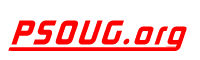Quick Search: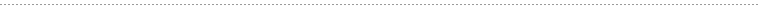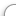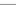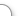CODE Oracle PL/SQL Code Library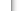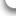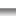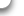JOBS Find Or Post Oracle JobsFORUM Oracle Discussion & ChatPHP Code: Awesome Date Time Conversion Kit Jump to: Select a Location PSOUG Home Page Oracle Code Library mySQL Code Library PHP Code Library JavaScript Code Library Oracle Terms & Definitions Oracle Error Codes PSOUG Community Blogs Oracle Jobs Board PSOUG Forum Oracle User Group Directory Free Oracle Magazines Online Learning Center PSOUG Presentations Advanced Code Search News and Events Sponsors Page Submit Code Contact UsSnippet Name: Awesome Date Time Conversion Kit

Description: Awesome Mysql date-time converter kit.

Real-life functions you can call to perform common date/time conversions between
MySQL datetime format, MySQL timestamp format and UNIX timestamp (i.e. seconds after epoch) with "human" output.

Comment: (none)

Language: PHP
Highlight Mode: PHP

```<?PHP

//we use UNIX's time specification as the base specification

FUNCTION mysql_datetime_to_human(\$dt){
\$yr=STRVAL(SUBSTR(\$dt,0,4));
\$mo=STRVAL(SUBSTR(\$dt,5,2));
\$da=STRVAL(SUBSTR(\$dt,8,2));
\$hr=STRVAL(SUBSTR(\$dt,11,2));
\$mi=STRVAL(SUBSTR(\$dt,14,2));
//              \$se=strval(substr(\$dt,17,2));

RETURN DATE("M/d/Y H:i", MKTIME (\$hr,\$mi,0,\$mo,\$da,\$yr))." MST";
}

FUNCTION mysql_timestamp_to_human(\$dt){

\$yr=STRVAL(SUBSTR(\$dt,0,4));
\$mo=STRVAL(SUBSTR(\$dt,4,2));
\$da=STRVAL(SUBSTR(\$dt,6,2));
\$hr=STRVAL(SUBSTR(\$dt,8,2));
\$mi=STRVAL(SUBSTR(\$dt,10,2));
//\$se=strval(substr(\$dt,12,2));

RETURN DATE("M/d/Y H:i", MKTIME (\$hr,\$mi,0,\$mo,\$da,\$yr))." MST";
}

FUNCTION mysql_timestamp_to_timestamp(\$dt){
\$yr=STRVAL(SUBSTR(\$dt,0,4));
\$mo=STRVAL(SUBSTR(\$dt,4,2));
\$da=STRVAL(SUBSTR(\$dt,6,2));
\$hr=STRVAL(SUBSTR(\$dt,8,2));
\$mi=STRVAL(SUBSTR(\$dt,10,2));
\$se=STRVAL(SUBSTR(\$dt,10,2));

RETURN MKTIME(\$hr,\$mi,\$se,\$mo,\$da,\$yr);
}

FUNCTION mysql_datetime_to_timestamp(\$dt){
\$yr=STRVAL(SUBSTR(\$dt,0,4));
\$mo=STRVAL(SUBSTR(\$dt,5,2));
\$da=STRVAL(SUBSTR(\$dt,8,2));
\$hr=STRVAL(SUBSTR(\$dt,11,2));
\$mi=STRVAL(SUBSTR(\$dt,14,2));
\$se=STRVAL(SUBSTR(\$dt,17,2));

RETURN MKTIME(\$hr,\$mi,\$se,\$mo,\$da,\$yr);
}

FUNCTION timestamp_to_mysql(\$ts){
\$d=GETDATE(\$ts);

\$yr=\$d["year"];
\$mo=\$d["mon"];
\$da=\$d["mday"];
\$hr=\$d["hours"];
\$mi=\$d["minutes"];
\$se=\$d["seconds"];

RETURN SPRINTF("%04d%02d%02d%02d%02d%02d",\$yr,\$mo,\$da,\$hr,\$mi,\$se);
}

FUNCTION timeleft(\$begin,\$end){
// for two timestamp format dates, returns the plain
// English difference between them.
// note these dates are UNIX timestamps

\$dif=\$end-\$begin;

\$years=INTVAL(\$dif/(60*60*24*365));
\$dif=\$dif-(\$years*(60*60*24*365));

\$months=INTVAL(\$dif/(60*60*24*30));
\$dif=\$dif-(\$months*(60*60*24*30));

\$weeks=INTVAL(\$dif/(60*60*24*7));
\$dif=\$dif-(\$weeks*(60*60*24*7));

\$days=INTVAL(\$dif/(60*60*24));
\$dif=\$dif-(\$days*(60*60*24));

\$hours=INTVAL(\$dif/(60*60));
\$dif=\$dif-(\$hours*(60*60));

\$minutes=INTVAL(\$dif/(60));
\$seconds=\$dif-(\$minutes*60);

\$s="";

//if (\$years<>0) \$s.= \$years." years ";
//if (\$months<>0) \$s.= \$months." months ";
IF (\$weeks<>0) \$s.= \$weeks." weeks ";
IF (\$days<>0) \$s.= \$days." days ";
IF (\$hours<>0) \$s.= \$hours." hours ";
IF (\$minutes<>0) \$s.= \$minutes." minutes ";
//if (\$seconds<>0) \$s.= \$seconds." seconds ";

RETURN \$s;
}

?>
```FreeOracle MagazineSubscriptionsand Oracle White Papers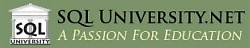SQL University.net courses meet the most demanding needs of the business world for advanced education in a cost-effective manner. SQL University.net courses are available immediately for IT professionals and can be taken without disruption of your workplace schedule or processes.

Compared to traditional travel-based training, SQL University.net saves time and valuable corporate resources, allowing companies to do more with less. That's our mission, and that's what we deliver.